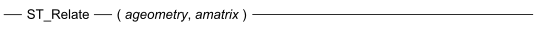# 16.20 - Syntax - Teradata Vantage NewSQL Engine

## Teradata Vantage™ Geospatial Data Types

prodname
vrm_release
16.20
created_date
March 2019
category
Programming Reference
featnum
B035-1181-162Kageometry
The other ST_Geometry value.
amatrix
A CHARACTER(9) value where each character corresponds to a cell in the DE-9IM pattern matrix.

The mapping for amatrix, as defined by SQL/MM Spatial, is as follows:

Position DE-9IM Cell
1 (Interior(SELF) ∩ Interior(ageometry)).ST_Dimension
2 (Interior(SELF) ∩ Boundary(ageometry)).ST_Dimension
3 (Interior(SELF) ∩ Exterior(ageometry)).ST_Dimension
4 (Boundary(SELF) ∩ Interior(ageometry)).ST_Dimension
5 (Boundary(SELF) ∩ Boundary(ageometry)).ST_Dimension
6 (Boundary(SELF) ∩ Exterior(ageometry)).ST_Dimension
7 (Exterior(SELF) ∩ Interior(ageometry)).ST_Dimension
8 (Exterior(SELF) ∩ Boundary(ageometry)).ST_Dimension
9 (Exterior(SELF) ∩ Exterior(ageometry)).ST_Dimension

Valid values for each character are: 'T', 'F', '0', '1', '2', and '*'.

The value specifies the set of acceptable values for an intersection at that given cell. The meaning is as follows.

Cell Value Intersection Set Results
'T' { 0, 1, 2 }
'F' { -1 }
'0' { 0 }
'1' { 1 }
'2' { 2 }
'*' { -1, 0, 1, 2 }